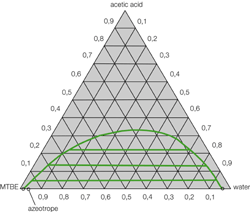# 0.90,10,80,70,30.60,40.50.50.60.30,70,2080,10.908 07 06 0,5 04 3 0,2 0,1

Question
192 views

The extraction of acetic acid from water is both industrially and environmentally significant. Methyl tert-butyl ether, or MTBE, has been identified as the best solvent for the extraction of acetic acid from water due to its higher selectivity, lower density, lower heat of vaporization, and lower cost. Below is the ternary phase diagram for the MTBE-acetic acid-water system. Note: for this problem, you can ignore the point that is labeled as an azeotrope.

In your system, you mix a 100 kg solution of 75 wt.% water/25 wt.% acetic acid with a stream of pure MTBE in an effort to extract the acetic acid. Two liquid phases form and reach equilibrium.

a. Draw and label your process flow diagram.

b. After the two liquid phases are formed, you measure the MTBE-rich phase and find that it contains 5 wt.% acetic acid. What is the full composition of this phase? What is the composition of the water-rich phase?

c. You want a higher acetic acid extraction in your MTBE-rich phase. How much MTBE must be added to the mixer to reach 14 wt.% acetic acid in your MTBE-rich phase?

d. Using the Gibbs phase rule, determine the degrees of freedom of a partially miscible ternary system (i.e. liquid-liquid equilibrium).help_outlineImage Transcriptionclose0.9 0,1 0,8 0,7 0,3 0.6 0,4 0.5 0.5 0.6 0.3 0,7 0,2 08 0,1 0.9 08 07 06 0,5 04 3 0,2 0,1 fullscreen
check_circle

Step 1

Part (a)

100 kg of the feed in stream 1 containing 75 wt% water (W) and 25% acetic acid (A). Stream 2 containing pure MTBE (M) is mixed with stream 1. The product streams 3 and 4 are in equilibrium with each other. The schematic flow diagram for the given process is drawn below.

Step 2

Part (b)

It is given that the MTBE rich stream 3 contains 5wt% acetic acid. Use the given ternary phase diagram as shown below to get the composition of the 2 product streams.

At point A, the composition of MTBE rich phase is shown. Draw a perpendicular line from the 0.05 of acetic acid line to point A. From this point A, draw perpendicular lines on MTBE and water lines and read from the plot the mass fractions of all the 3 species in the stream 3 (MTBE rich phase). Therefore,

x3(A) = 0.05

x3(W) = 0.02

x3(M) = 0.93

Now, draw a line parallel to the ties line given from point A to point B. This is the equilibrium line between the two product streams. From this point B, draw perpendicular lines on MTBE, acetic acid and water lines and read from the plot the mass fractions of all the 3 species in the stream 4 (water rich phase). Therefore,

X4(A) = 0.02

X4(W) = 0.92

X4(M) = 0.06

Step 3

Part (c)

For 14 wt% acetic acid in the MTBE rich stream 3, use the given ternary phase diagram as shown below to get the composition of the 2 product streams.

At point A, the composition of MTBE rich phase is shown. Draw a perpendicular line from the 0.14 of acetic acid line to point A. From this point A, draw perpendicular lines on MTBE and water lines and read from the plot the mass fractions of all the 3 species in the stream 3 (MTBE rich phase). Therefore,

x3(A) = 0.14

x3(W) = 0.03

x3(M) = 0.83

No...

### Want to see the full answer?

See Solution

#### Want to see this answer and more?

Solutions are written by subject experts who are available 24/7. Questions are typically answered within 1 hour.*

See Solution
*Response times may vary by subject and question.
Tagged in

### Chemical Engineering